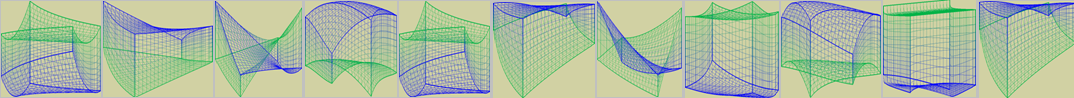### Principle##### New Square Method

◆ "New square method" is an improved method of "least square method", and "least square method" is a special case of "new double multiplication". It can replace all the previous regression analysis algorithms. It not only calculates the constants and coefficients of the model, but also calculates the power value in the model variables, making the regression analysis simpler and the results more accurate.

◆ "New square method" in regression processing of two-dimensional data, a variable can derive multiple elements with different powers of the same variable. When processing multi-dimensional data, considering the interaction of different variables, multiple elements can be derived to make the regression analysis results more accurate.

Details##### Dynamic local analysis

◆ "Dynamic local analysis" is an algorithm for big data analysis.

◆ Big data has many dimensions, a wide range and a large amount of data, and it is difficult to find the mathematical relationship between them with the "New square method". For the first problem, we developed the "Dynamic local analysis" method.

◆ "Dynamic local analysis" is to screen the original historical data and eliminate unreasonable samples to obtain N groups of samples. During sample analysis, find the k (k can be set as 10) sample closest to the sample in N groups of samples, use k sample to model, and substitute the sample data into the model for calculation, and the calculation results meet the accuracy requirements.

Details

### Demonstration##### ◆ The factors and results are complete samples, and the characteristics of the samples need to be checked. When the factor dimension is greater than 50, it should be reduced to 50 dimensions.Details<=50 Demo>50 Demo##### ◆ All factors and results are in two files respectively, and the characteristics of the sample are checked at the same time; When the factor is greater than 50 dimensions, it must be artificially reduced to 50 dimensions.Details<=50 Demo>50 Demo##### ◆ All factors are in one file, the result is a single data, and the characteristics of the sample are checked at the same time; When the factor is greater than 50 dimensions, it must be artificially reduced to 50 dimensions.Details<=50 Demo>50 Demo##### ◆ Each factor and result are in one file, and the characteristics of the sample are checked at the same time; When the factor is greater than 50 dimensions, it must be artificially reduced to 50 dimensions.Details<=50 Demo>50 Demo##### ◆ The system can match the data into new samples with the growth of time. The merging of new and old samples can make the samples more perfect. Using the merged samples will improve the calculation accuracy.DetailsDemo##### ◆ Samples and dimensions have outliers or do not comply with system laws. One dimension is derived from another dimension, so these samples or dimensions should be eliminated.DetailsDemo##### ◆ The size span and order of magnitude of the data parameters of the system are also different. The order of magnitude of each dimension can be the same through mathematical methods, or the weight can be given according to experience.DetailsDemo##### ◆ After sample preprocessing, adopt different methods and establish different mathematical algorithms according to the situation. Different methods can be used for calculation, which can support each other.DetailsDemo##### ◆ The system is not unique, but there are differences between systems and the data obtained are also different. Use mathematical methods to find out the gap and let the system method be used on another system.DetailsDemo##### ◆ During calculation, one sample can be calculated separately, one sample can calculate multiple results, and multiple samples can calculate multiple results, which can be calculated continuously in real time.DetailsDemo##### ◆ Set the upper and lower limits of samples and results. If the limit is exceeded during calculation, the parameters shall be adjusted in time to ensure the normal operation of the system.DetailsDemo### COMMUNICATE#### BDA

Language: Simplified Chinese

Size: 37.9 MB

Description:Big data analysis system

(BDA) is a collection version of

quality control chart (QCC), data

regression analysis (DRS) and system

process analysis(SPA).COMMUNICATE#### QCC

Language: Simplified Chinese

Size: 27.3 MB

Description:Quality control chart

(QCC) is a quality control tool

prepared according to the

national standard of the people's

Republic of China "GB_T_4091-2001".COMMUNICATE#### DRS

Language: Simplified Chinese

Size: 34.1 MB

Description:Data regression analysis

(DRS) was prepared according to

"New Square Method". It makes the

regression calculation of one-

dimensional and multi-dimensional

linear and nonlinear data simpler

and the result more accurate.COMMUNICATE#### SPA

Language: Simplified Chinese

Size: 36.8 MB

Description:System process analysis

(SPA) is a tool to analyze the data

of various relationships within a

system, establish mathematical

relationships and calculate. It

is widely used in practice.COMMUNICATE